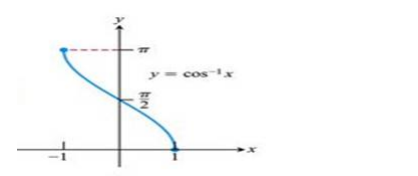# Mark the tick against the correct answer in the following:

Question:

Mark the tick against the correct answer in the following:

Domain of $\cos -1 \times$ is

A. $[0,1]$

B. $[-1,1]$

C. $[-1,0]$

D. None of these

Solution:

To Find: The Domain of $\cos ^{-1}(x)$

Here,the inverse function of $\cos$ is given by $\mathrm{y}=\mathrm{f}^{-1}(x)$

The graph of the function $y=\cos ^{-1}(x)$ can be obtained from the graph of

$Y=\cos x$ by interchanging $x$ and $y$ axes.i.e, if $(a, b)$ is a point on $Y=\cos x$ then $(b, a)$ is the point on the function $y=\cos ^{-1}(x)$

Below is the Graph of the domain of $\cos ^{-1}(x)$From the graph, it is clear that the domain of $\cos ^{-1}(x)$ is $[-1,1]$